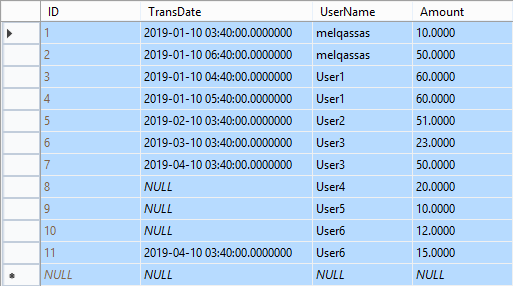# Get the first record added per day group by the user in SQLIn the previous post Get the last record added per day group by the user in SQL I explained How to get the last entry for each user per day.

In this post, I am gonna to explain

How to get the first record added per day for each user in SQL.

## Get the first entry added per day for each user in SQL

Consider you have a [Transaction] table to save multiple transactions for different users daily!

The [Transaction] table structure is simple like the below one:

• ID.
• Transaction Date.
• Amount…etc.

In this table, the user add multiple transactions per day! and you would like to know what’s the first record added per day for each user!

In this post, I am gonna to explore two different queries to get the first entry for each user per day as the following:

### Using Inner Join

#### Steps

1. Get all required field from the main table [Transaction].
2. Get all records aggregated with Minimum function on the [TransDate] grouped by [UserName] field.
3. Use “WITH” clause to give the above query a block name (MinTransTable).
4. Perform inner join between main [Transaction] table and [MinTransTable].
##### Get all required field

Get all required field from the main table [Transaction].

```SELECT [Transactions].UserName,
[Transactions].TransDate,
[Transactions].Amount
FROM [dbo].[Transactions]```
##### Get all records aggregated by Min date

Get all records aggregated with Minimum function on the [TransDate] grouped by [UserName] field.

```SELECT [UserName],
Min[TransDate]) AS MinDate
FROM [dbo].[Transactions]
##### Use “WITH” clause

Use “WITH” clause to give the above query a block name (MinTransTable).

```WITH MinTranstable AS
min([TransDate]) AS MinDate
FROM [dbo]. [Transactions]
##### Perform inner join

Perform inner join between main [Transaction] table and [MinTransTable].

```WITH MinTranstable AS
min([TransDate]) AS MinDate
FROM [dbo]. [Transactions]
[Transactions].TransDate,
[Transactions].Amount
FROM [dbo].[Transactions]
AND [Transactions].TransDate = MinTranstable.MinDate```

The final result would be like

You may be also interested to read SQL Server: Get the Detailed Information Via SERVERPROPERTY.

### Using row_number() function

Firstly, Let’s explain what’s the row_number() function?

• It’s a function that assigns a sequential integer to each row within a partition of a result set.
• You can use it with “PARTITION BY” to divide the result set into partitions per specific column, actually, it’s an optional part, if you don’t use it, the function will treat all rows of the query result set as a single group.

#### Steps

1. Get all rows from [Transaction] table with a sequential row number for each “[UserName]” ordered by “[Transaction Date]” Ascending.
2. Use the “WITH” clause to give the above query a block name (MinTransTable).
3. Get all records filtered by the sequential number ID= 1 and the [Transaction date] is not null.
##### Get all rows from [Transaction] table

Get all rows from [Transaction] table with a sequential row number for each “[UserName]” ordered by “[Transaction Date]” Ascending.

```SELECT row_number() over(PARTITION BY UserName ;
ORDER BY TransDate ASC) AS ID,
[TransDate],
[Amount]
FROM [dbo].[Transactions] )```
##### Use the “WITH” clause

Use “WITH” clause to give the above query a block name (MinTransTable)

```WITH MinTransTable AS
ORDER BY TransDate ASC) AS ID,
[TransDate],
[Amount]
FROM [dbo]. [Transactions])```
##### Get all filtered records

Get all records filtered by the sequential number ID= 1 and the [Transaction date] is not null.

```WITH MinTransTable AS
ORDER BY TransDate ASC) AS ID,
[TransDate],
[Amount]
FROM [dbo]. [Transactions])
SELECT *
FROM MinTransTable
WHERE MinTransTable.ID = 1
AND MinTransTable.[TransDate] IS NOT NULL```

The final result would be as the following:

#### Which Query is the best one?

Although the two queries generate the same result, but as per my experiment using actual execution plan, the row_number() query is faster than the inner join query!

###### Applies To

SQL Server 2012 and later.